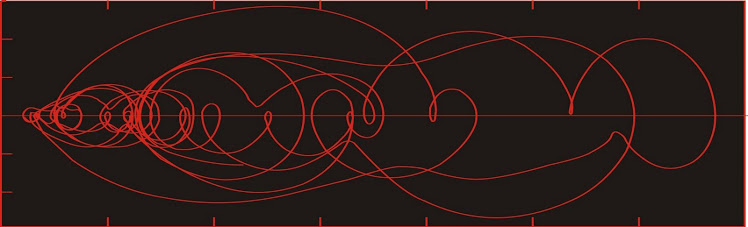## Dust flux, Vostok ice coreTwo dimensional phase space reconstruction of dust flux from the Vostok core over the period 186-4 ka using the time derivative method. Dust flux on the x-axis, rate of change is on the y-axis. From Gipp (2001).

## Sunday, March 13, 2011

### Tsunami dynamics--why they can cross an ocean

Friday's earthquake off the coast of Japan reminds us of the power of water.

But first, a little animation of a model of the tsunami.

Here is a model of amplitude of wave height as posted by NOAA.

This model is constructed first from knowledge of the motion of the earthquake, which can be seen here.

The beachballs in the figure above give you a sense of the motion of the earthquake. To interpret them, imagine a beachball divided into four longitudinal quadrants. One pair of opposing quadrants is black, the other pair is white. The black refers to the portion of the globe where the first motion of the ground after the earthquake is compressive (i.e., the ground is pushed away from the focal point). The white areas represent the areas on the globe where the first motion is extensional (i.e., the ground is pulled toward the focal point).

In order to divide the beachball into four quadrants, you have two intersecting planes. In the diagram above, we can see that of the two planes, one must be approximately vertical, and the other is nearly horizontal. One of these planes is the fault plane--the plane along which the earth has broken. In the diagram above, we are not given enough information to tell which one it is, but additional information on the USGS website allows us to tell it was the nearly horizontal plane.

The other plane is perpendicular to the direction of motion along the fault plane. Knowing that the nearly horizontal plane is the fault plane, we can tell that the direction of motion is perpendicular to the nearly vertical plane, which is oriented approximately NNE-SSW. The direction of motion is therefore either north of west, or south of east. Since the compressive motion is on the west side, we know the motion was towards the west.

Notice the thin arc of white around the Harv and CPPT solutions. The ground in the black has moved upward/outward from the focal point, and the white areas have moved toward the focal point. Hence Japan, to the west of the fault has moved towards it, but much of the seafloor between Japan and the focus has moved upwards, spawning the tsunami.

The tsunami starts off from the earthquake epicentre and flows outwards. In order to model what happens next, you need a model of the topography of the ocean basin.

The velocity of the tsunami is a function of water depth--the deeper the water the faster it goes. When the wave hits shallower water it slows down, and piles up as the faster moving water behind it catches up to it All of those little and islands and seamounts will diffract some of the energy (in doing so they act like new, but smaller sources of radial waves). Either the islands or the topography may act to focus the energy of the wave along some portions of the wave front, explaining how central California was hit by higher waves than areas either north or south of it.

The key to the energy transmission on a global scale is the geometry of the source. If you throw a pebble into a pond, the ripples you get have the form of a series of expanding circles.

Consider the portion of the ripple outlined in red (between the two yellow lines). Let us say that the quantity of energy represented in that portion of the ripple is a. As the ripple expands, the amount of energy in the ripple remains the same (it actually declines due to friction and internal factors). However as the length of the red arc increases as the ripple expands, the amount of energy per unit length of the wave declines with distance travelled (in addition to friction and other losses).

An earthquake occurs along a plane. The motion does not occur along the plane everywhere at once, but propogates at a finite speed.

Diagram showing both magnitude of motion along the fault (colours) and the length
of time before motion was initiated at points along the fault (contours). Source here.

Thus instead of a point source for the wave, you have a line source (we are only considering the ocean surface at this point). The effect of a line source makes a tremendous difference in energy propagation.

Note here that as the waves propagate, the lengths of the red arcs stay constant. Thus there is no loss of energy to expansion of the wavefront over parts of the tsunami (not the case at the ends in the diagram above). There are still losses due to friction and internal dissipation.

Here is a screen capture of the NOAA model (from the model above).

Right at the moment of this capture, the wavefront heading towards South America is nearly linear, meaning there is little loss of energy as the wave flows. Where the front is curved, energy is declining dramatically.